# Equivariant cohomology

Jump to: navigation, search

A cohomology that takes the action of some group into account. More precisely, an equivariant cohomology in the category of-spaces (that is, topological spaces on which the continuous action of a groupis defined) and equivariant mappings is a sequence of contravariant functors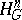(taking values in the category of Abelian groups) and natural transformations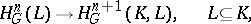with the following properties: a) equivariantly-homotopic mappings of pairs induce identity homomorphisms of the groups; b) an inclusion of the form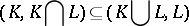induces an isomorphismand c) for every pair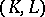the following cohomology sequence is exact: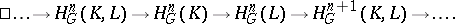Letbe a universal-fibration and let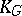be the space associated with the universal fibre spacewith fibre(that is, the quotient space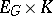under the action ofgiven by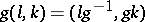). Then the functors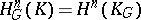yield an equivariant cohomology theory; here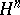is an arbitrary cohomology theory.

For any fixed groupthe collection of groupstogether with all possible homomorphisms induced by inclusions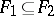of subgroups ofis usually called the system of coefficients for the-theory. In some cases the functorsare uniquely defined by their systems of coefficients (for example, whenis finite and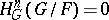for).